# 日志OLAP：在SQL中使用UDF, lambda函数使用案例

+关注继续查看

## 场景

__source__:  11.164.232.105
__tag__:__hostname__:  vm-req-170103232316569850-tianchi111932.tc
__topic__:  TestTopic_4
array_column:  [1,2,3]
double_column:  1.23
map_column:  {"a":1,"b":2}
text_column:  商品

## lambda函数对array类型的数据进行求均值

* | select  array_column,  reduce( cast(  json_parse(array_column) as  array(bigint))  , CAST(ROW(0.0, 0) AS ROW(sum DOUBLE, count INTEGER))  ,  (s,x) -> cast(row( x+ s.sum,  s.count+1) as ROW(sum double, count INTEGER)), s -> IF(s.count = 0, NULL, s.sum / s.count))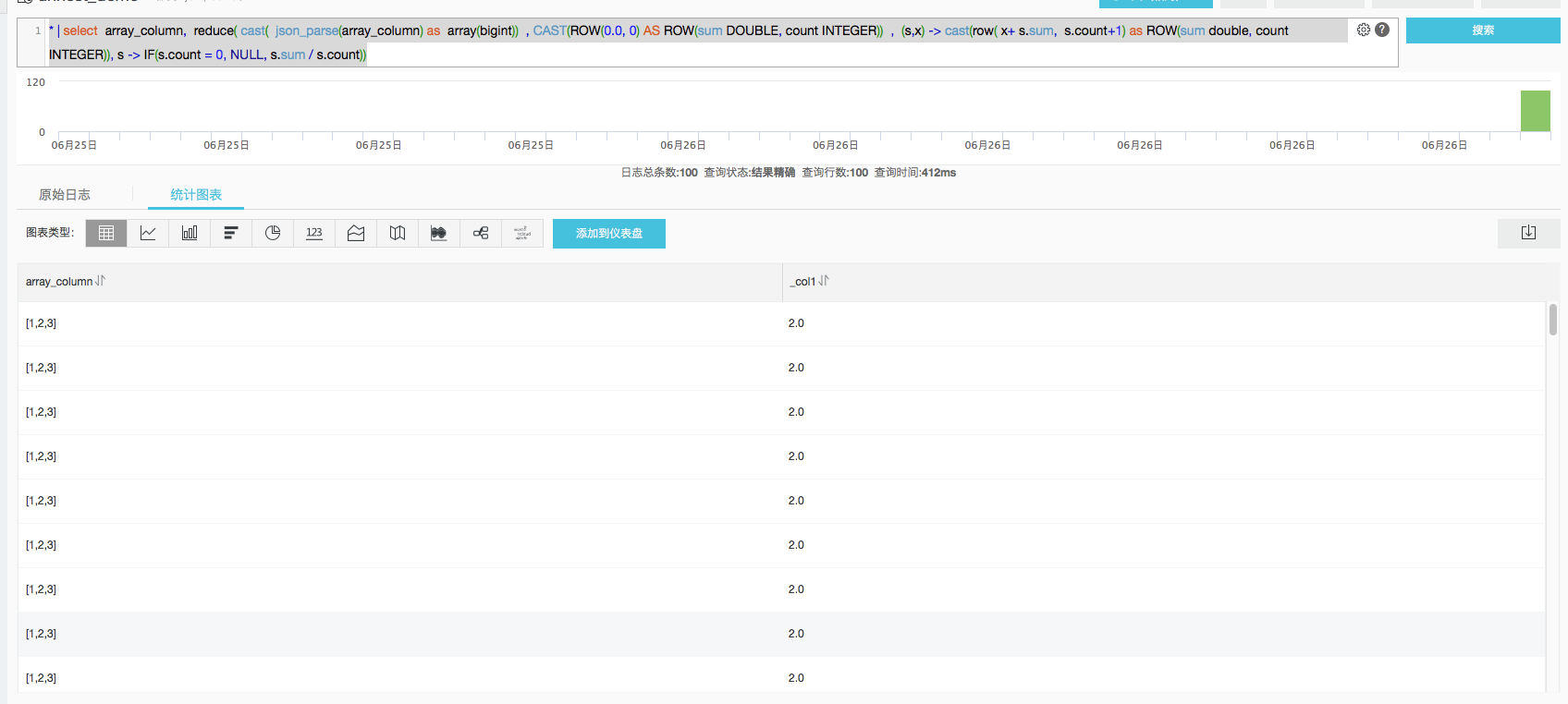reduce 函数的具体语义参考语法文档。参数分为四部分

1. cast( json_parse(array_column) as array(bigint)) 表示输入的数组数据
2. CAST(ROW(0.0, 0) AS ROW(sum DOUBLE, count INTEGER)) 定义起始状态为一个复杂的row类型，分别记录sum和count
3. 对每一个元素，计算累加值，(s,x) -> cast(row( x+ s.sum, s.count+1) as ROW(sum double, count INTEGER)) s代表已经有的状态，x代表新输入的元素，计算结果通过cast强制定义为row类型
4. 最后对最终状态，计算avg值，s -> IF(s.count = 0, NULL, s.sum / s.count)。s代表最终状态。

## 对所有行的array元素求avg：

* |  select  sum(rows.sum ) / sum(rows.count)  from(
select  array_column,  reduce( cast(  json_parse(array_column) as  array(bigint))  , CAST(ROW(0.0, 0) AS ROW(sum DOUBLE, count INTEGER))  ,  (s,x) -> cast(row( x+ s.sum,  s.count+1) as ROW(sum double, count INTEGER)), s -> s)  as rows from log
)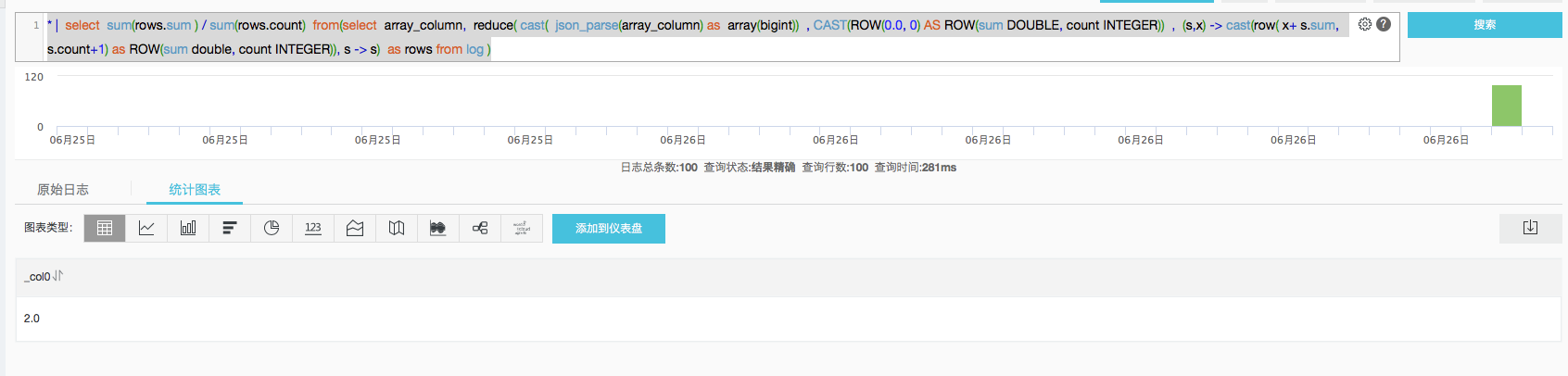Java Lambda 的秘密 ｜ Java Debug 笔记
Java Lambda 的秘密 ｜ Java Debug 笔记
31 0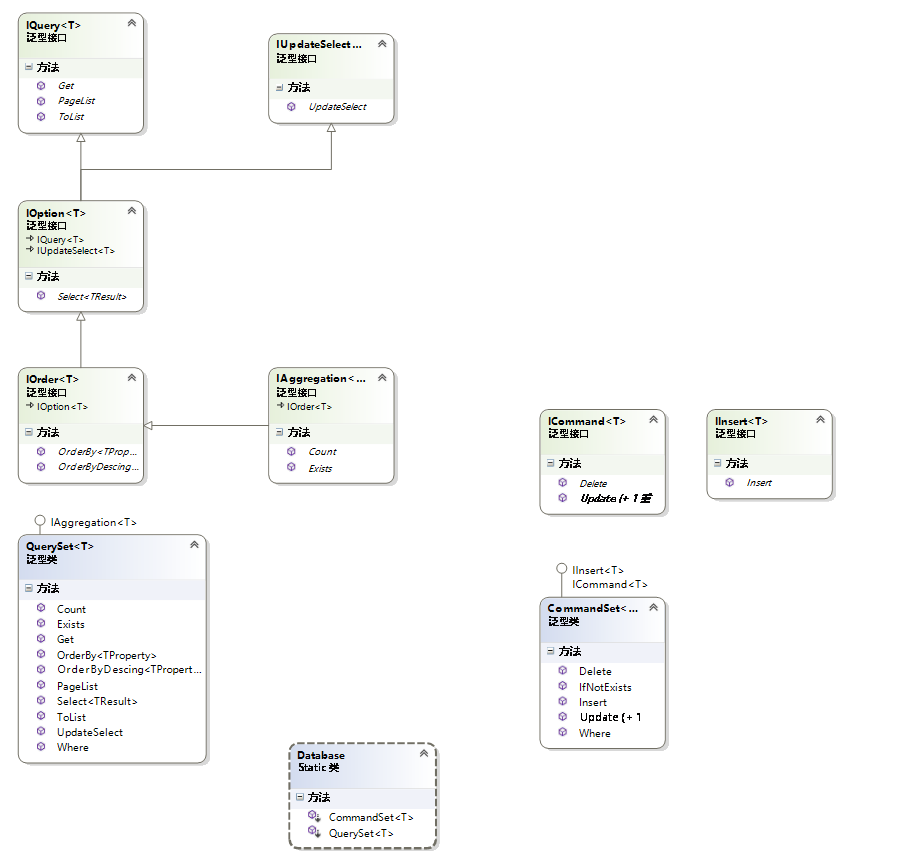27 056 0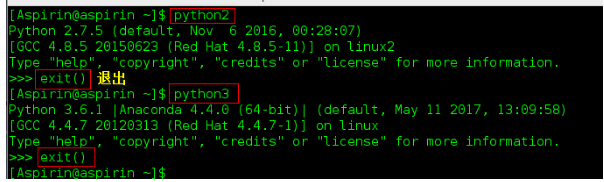CentOS 7-(64位)系统下安装Anaconda（Python3.x）及pip使用
CentOS 7-(64位)系统下安装Anaconda（Python3.x）及pip使用
34 07706 0815 0php5.6完全yum安装LAMP环境并加载fastcgi模块
1268 0c/c++_Lua交互----关于Lua中table类型的使用实例
lua中的复合类型 只有table 类型,你可以当做任意容器使用  ,比如 数组    PHP中的关联数组  C++中的 std::map 等等  而且提供了很方便的使用 下面是lua中 table类型的使用 c++加载代码  #include "string.
803 0C++ 仿函数/函数指针/闭包lambda
3647 0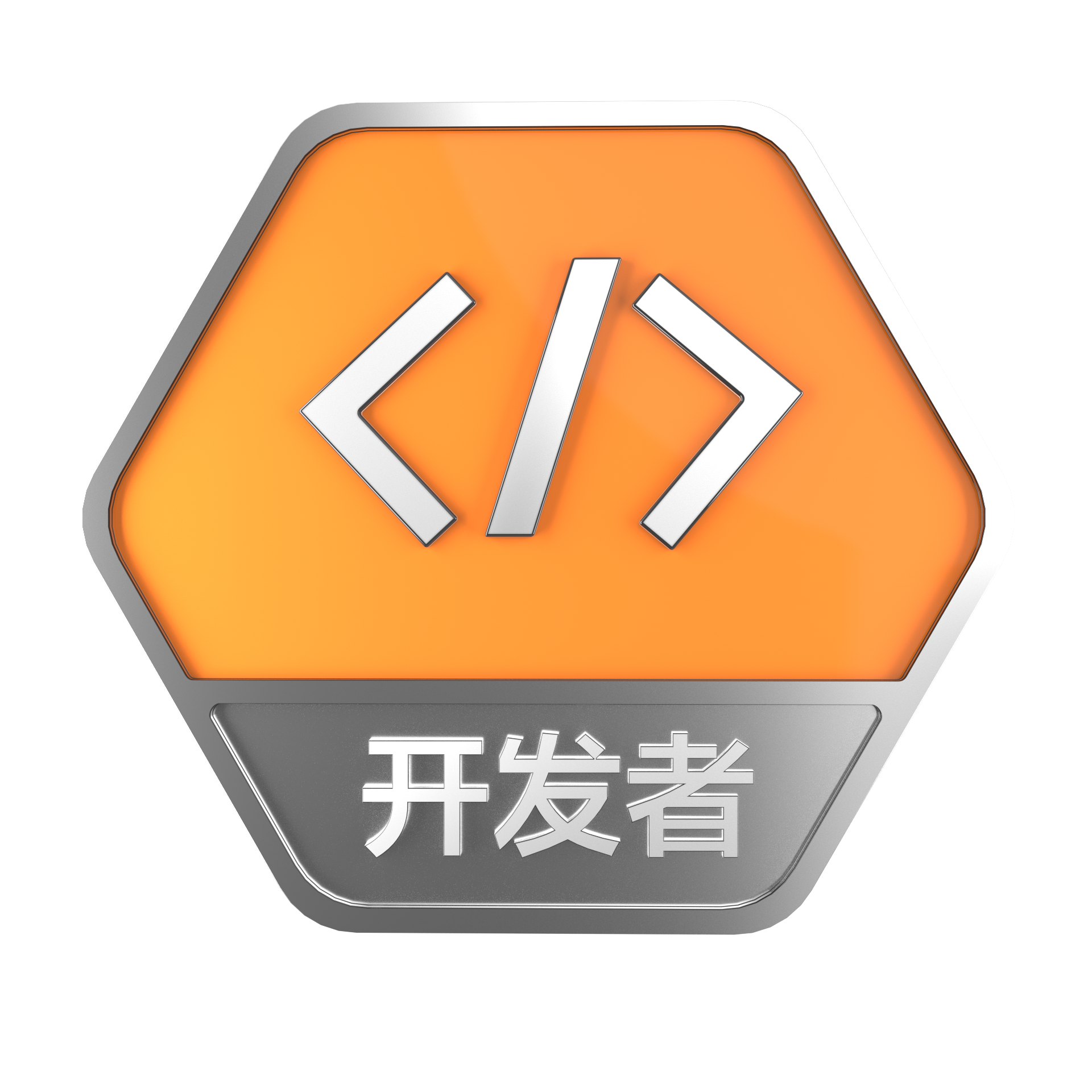+关注

104

2

+ 订阅